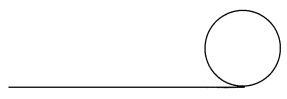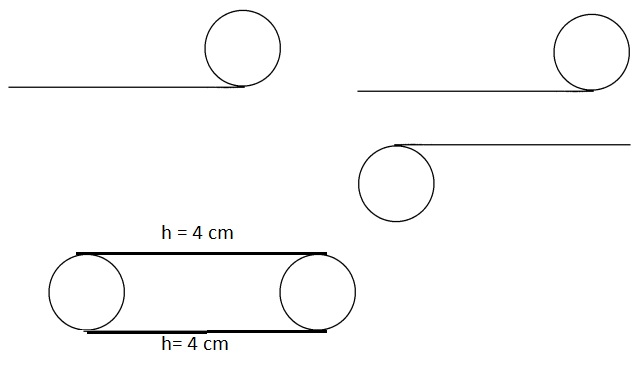# Math in Focus Grade 5 Chapter 14 Practice 2 Answer Key Cylinder, Sphere, and Cone

This handy Math in Focus Grade 5 Workbook Answer Key Chapter 14 Practice 2 Cylinder, Sphere, and Cone provides detailed solutions for the textbook questions.

## Math in Focus Grade 5 Chapter 14 Practice 2 Answer Key Cylinder, Sphere, and Cone

Identify each solid shape.

Question 1.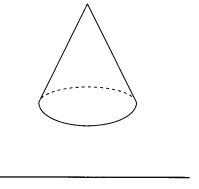Explanation:
Given solid shape is cone as it has 2 faces and 1 vertices.

Question 2.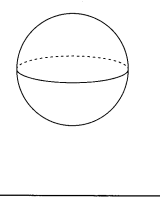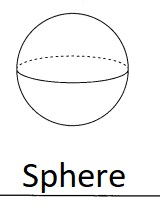Explanation:
Given solid shape is sphere as it has 1 face, 0 edge and 0 vertices.

Question 3.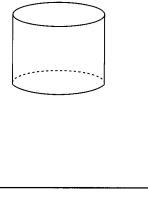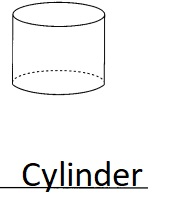Explanation:
Given solid shape is cylinder as it has 3 faces, 2 edges and 0 vertices.

Question 4.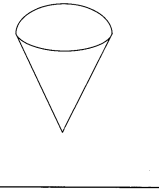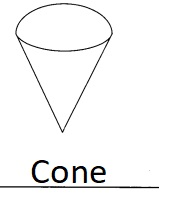Explanation:
Given solid shape is cone as it has 2 faces and 1 vertices.

Identify the shape of the base of each solid shape.

Question 5.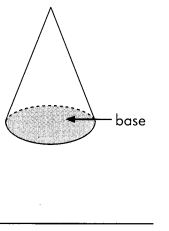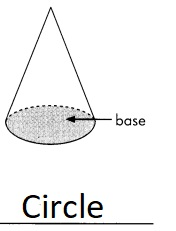Explanation:
The base of the cone is a flat face which is a circle.

Question 6.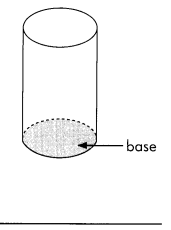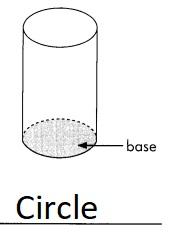Explanation:
A cylinder is a three-dimensional solid that holds two parallel
bases joined by a curved surface, at a fixed distance.
Bases are normally circular in shape (like a circle).

Describe the labeled surface of each solid shape.

Question 7.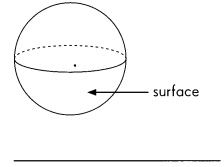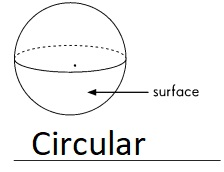Explanation:
A sphere is a three dimensional figure that is the set of all points
equidistant from a fixed point called the center of circular shape.

Question 8.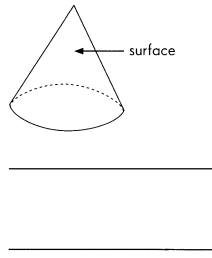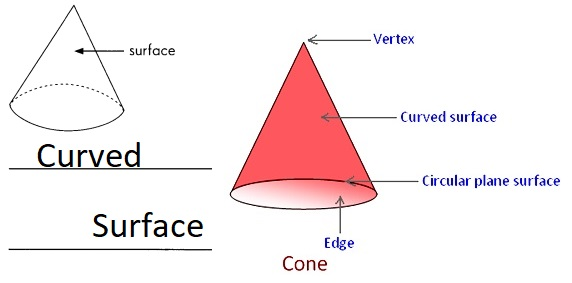Explanation:
Given cone is pointing towards curved surface of cone as shown above.

Question 9.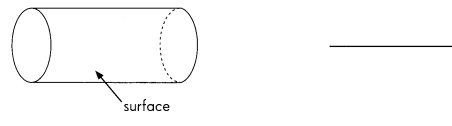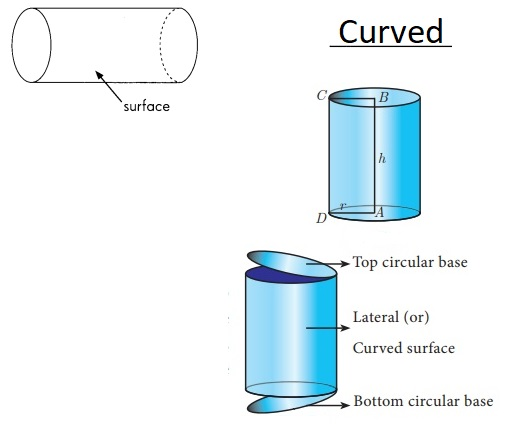Explanation:
Given cylinder is pointing towards curved surface of cylinder as shown above.

Complete.

Question 10.
The height of a cylinder is 4 centimeters. Complete the net of this open cylinder.